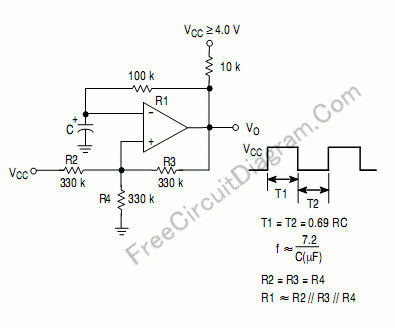# Square Oscillator Using Comparator: The Circuit Schematic Diagram and The FormulaUsing a single supply quad comparator LM339, LM239, LM2901, LM2901V, NCV2901, or MC3302, we can build a square-wave oscillator with simple circuit and formula. Here is the schematic diagram:This circuit is suitable if you need  many square-wave oscillators in one circuit, since the quad packaged comparator provide four comparators in one single  integrated chip. [circuit’s schematic diagram source: Motorola Application Notes]